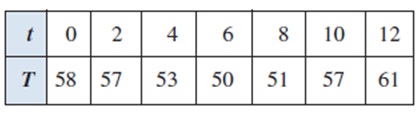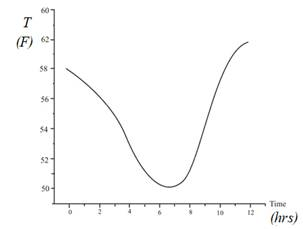# Given graph,### Precalculus: Mathematics for Calcu...

6th Edition
Stewart + 5 others
Publisher: Cengage Learning
ISBN: 9780840068071### Precalculus: Mathematics for Calcu...

6th Edition
Stewart + 5 others
Publisher: Cengage Learning
ISBN: 9780840068071

#### Solutions

Chapter 2.1, Problem 82E
To determine

## Given graph,Expert Solution

The Graph of the function as shown below:### Explanation of Solution

Given information:

The follow graph shows the daily temperature reading T(in F0) recorded every 2 hour from midnight to noon in Atlanta, Georgia, March, 18, 1996

Using the given table, draw the Graph of the function as shown below:### Have a homework question?

Subscribe to bartleby learn! Ask subject matter experts 30 homework questions each month. Plus, you’ll have access to millions of step-by-step textbook answers!